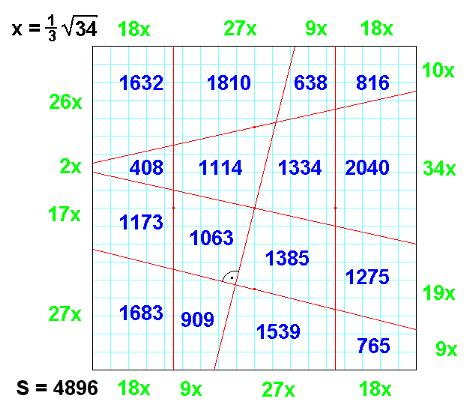Observations on 4x4 Area Magic Squares with Vertical Lines
© H.B. Meyer         classical 4x4 magic squares         magic squares and cubes         Seite in deutscher Sprache

 28 26 24 22 13 21 29 37 40 30 20 10 19 23 27 31

is a 4x4 Area Magic Square with Vertical Lines (VL-AMS):
- the 16 entries are different natural numbers,
- the 4 rows, the 4 columns and the 2 diagonals sum up to the same result (=100).
- there exists a geometric square (with side length 20 here), which can be divided into 16 trapezoids by 3 vertical and 3 skew straight lines,
such that every VL-AMS entry is exactly the area of the corresponding trapezoid.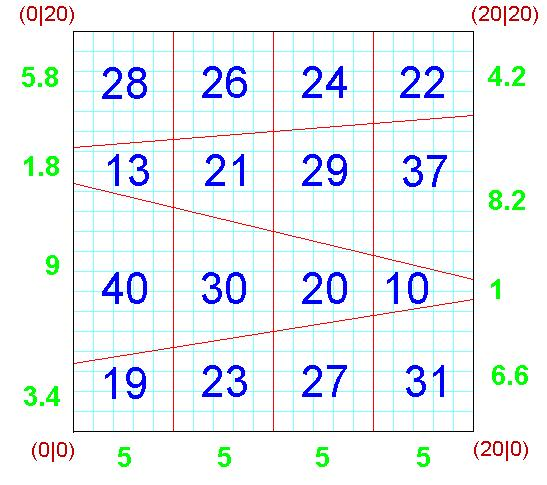The entry set T={10,13,19,20,21,22,23,24,26,27,28,29,30,31,37,40} is a symmetric set, i.e.: for every x in T the number N+a-x also belongs to T; here N=40 denotes the greatest and a=10 the smallest element of T.

Purpose of this website   4x4 VL-AMS are considered. Which and how many VL-AMS do exist and which structure do they and their entry sets have.

Remark  The concept "Linear Area Magic Square" is an idea due to William Walkington; the first VL-AMS (in a rotated version) was found by Walter Trump; many of the considerations described here are owed to him.

Observation 1   If q denotes the side length of a geometric square with lower left vertex in (0|0), belonging to any VL-AMS, then the 3 non-vertical separation lines run through the points (q/2|3q/4) resp. (q/2|q/2) resp. (q/2|q/4).
In in every row of the VL-AMS neighbouring entries have the same difference.
 Observation 2   For every VL-AMS with smallest entry a und greatest entry N the magic sum is 2(N+a). There are 8 pairs of entries with sum N+a. If both entries of such a pair are connected by a line, then the "connection figure" of the VL-AMS is formed: the VL-AMS is a magic square of Dudeney Typ VI. Reflection at a vertical or horizontal central line or a 180 degree rotation of any VL-AMS results to a VL-AMS again.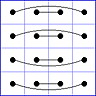Observation 3  Every VL-AMS can be described by 3 parameters s,t and a as follows: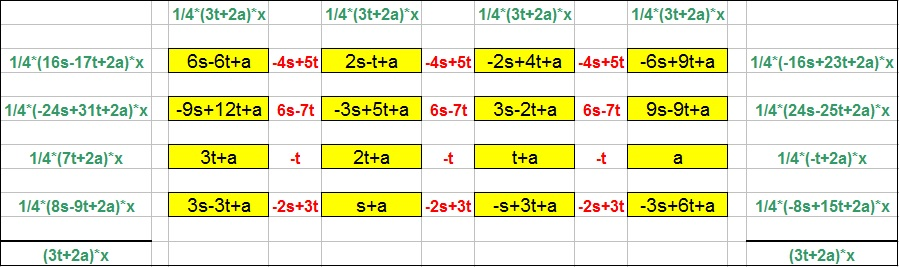The yellow marked terms are the VL-AMS entries, the red expressions are the differences of rowwise neighboured entries and the green terms each give the side length of a trapezoid on the left or right border of the geometric square. It is not difficult to prove this parametrisation, using linear algebra.
The geometric square's side length is q = (3t+2a)*x, with unit grid length x = √(8/(3t+2a)). The magic sum is S = 6t+4a, so q = 2*√S; if S is a square number, then all 16 trapezoids have rational side length'.
The slopes of the 3 non vertical separation lines are m1 = (8s-10t)/(3t+2a), m2 = (-4s+4t)/(3t+2a), and m3 = (-4s+6t)/(3t+2a) with m1 + m2 + m3 = 0.
The above example has parameters s=13, t=10, a=10.

Observation 4  When the entry 6s-6t+a is compared with 3s-3t+a and with 9s-9t+a it follows, that 6s-6t+a cannot be the smallest VL-AMS entry.
Because of rotation and reflections the smallest entry cannot be a vertex-entry. Therefore without loss of generality, it may be assumed that the parameter a is the lowest entry and then N=3t+a is the greatest one.

Observation 5  All VL-AMS entries as well as every side length' (green) of any trapezoid must be positive, this requires the conditions:
(1)     0 < t < s < 4/3*t < 8/3*a.

Observation 6  All yellow marked number terms of the parametrisation above have to evaluate to pairwise different values, this will be ensured by the conditions
(2)     n*s ≠ (n+1)*t for n = 4,5,6,7,8,9 and n*s ≠ (n+2)*t for n = 7,9,11.
According to inequalities (1) and (2) the smallest possible parameter t is t=10 and therefore s=11 and a=6 are the smallest ones; they lead to the smallest possible magic sum S=84.

Observation 7  When 1 is added to every entry of a VL-AMS, the result will be another VL-AMS, as well as if every entry is multiplied with a positive integer factor.
Any linear transfomation VL-AMS2 = r * VL-AMS1 + b with integer r and b produces a VL-AMS2 from a VL-AMS1.
A VL-AMS is called fundamental, if it cannot be obtained from another one by any such transformation. There exist 720 VL-AMS with magic sum S ≤ 200, among them are 50 fundamental ones.
A file containing these named VL-AMS can be found here, read the entries rowwise from left above to right below.

Observation 8  There also exist linear area 4x4 magic squares with only 2 vertical lines ("V2L-AMS"):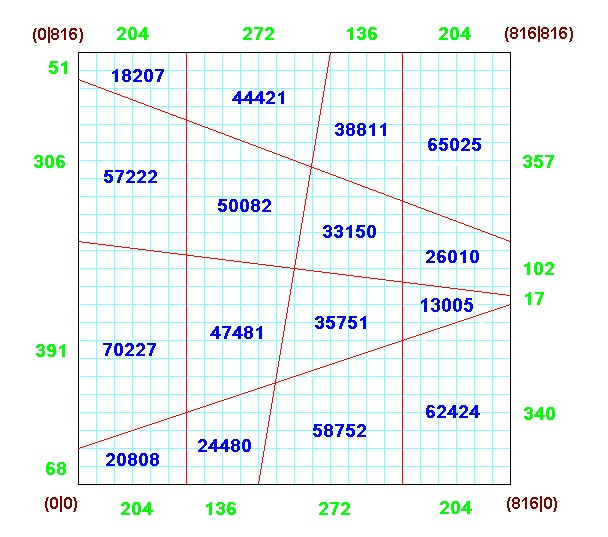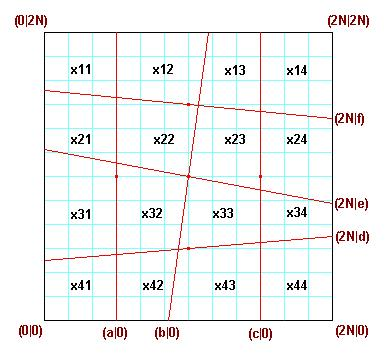This example with magic sum 8162/4 is of Dudeney type VI, too. Here all shown numbers are multiples of 17. It was found with a search for natural numbers b,d,e,f, b≠N, on a 2N x 2N grid, such that the x11,x12,....x44 initially represent a magic square with rational entries, which is the case, when x11+x22+x33+x44 = x14+x23+x32+x41 = N2 with all 16 entries different.
Then the main denominator HN for x11,x12,...,x44 was determined and every entry multiplied with HN2. Using reflections or 180°rotation one can manage b<N and d+N≤f. A file containing such magic squares from N=24 to N=96 can be found here. The (already with HN2 multiplied entries) have to be set in 4 rows from left above to right below.
For any given 4x4 area magic square with 2 vertical lines the numbers d,e and f can be determined easily, f.i.: d = (14x44-2x41)/(6N); a formula for b is b = N/2 + (-x11+12x13-5x14-x21+12x23-5x24)/(6N).
There are also V2L-AMS' where the line defined by (b|0) stands perpendicular to the line through (2N|d), this is the case, when 2b-2d = N.
See example, derived from N=36, b=27, d=9, e=28, and f=62: